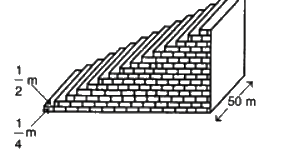# Ex.5.4 Q5 Arithmetic progressions Solutions - NCERT Maths Class 10

Go back to  'Ex.5.4'

## Question

A small terrace at a football ground comprises of $$15$$ steps each of which is $$50\, \rm{m}$$ long and built of solid concrete. Each step has a rise of \begin{align}\frac{1}{4}\,\rm{m} \end{align} and a tread of \begin{align}\frac{1}{2}\,\rm{m} \end{align} (See figure) calculate the total volume of concrete required to build the terrace.Video Solution
Arithmetic Progressions
Ex 5.4 | Question 5

## Text Solution

What is Known?

$$15$$ steps each of which is $$50 \,\rm{m}$$ long and each step has a rise of \begin{align} \frac{1}{4}\,\rm{m} \end{align} and a tread of \begin{align} \frac{1}{2}\,\rm{m} \end{align}

What is Unknown?

Total volume of concrete required to build the terrace.

Reasoning:

Sum of the first $$n$$ terms of an AP is given by \begin{align} {S_n} = \frac{n}{2}\left[ {2a + \left( {n - 1} \right)d} \right] \end{align}

Where $$a$$ is the first term, $$d$$ is the common difference and $$n$$ is the number of terms.

Steps:

From the figure, it can be observed that

Height of $$1^{st}$$ step is \begin{align}\frac{1}{4} \,\rm{m} \end{align}

Height of $$2^{nd}$$ step is \begin{align} \left( {\frac{1}{4} + \frac{1}{4}} \right) \,\rm{m} = \frac{1}{2} \,\rm{m} \end{align}

Height of $$3^{rd}$$ step is \begin{align} \left( {\frac{1}{2} + \frac{1}{4}} \right)\,\rm{m} = \frac{3}{4} \,\rm{m} \end{align}

Therefore, height of the each step is increasing by \begin{align} \frac{1}{4} \,\rm{m} \end{align}  length $$50 \,\rm{m}$$ and width (tread) \begin{align} \frac{1}{2} \,\rm{m} \end{align} remain the same for each of the steps.

Volume of Step can be considered as Volume of Cuboid= Length $$\times$$ Breadth $$\times$$ Height

Volume of concrete in $$1^{st}$$ step \begin{align}\!=\!50m \!\times\! \frac{1}{2} \,\rm{m} \!\times\! \frac{1}{4} \,\rm{m} \!=\! 6.25\,{m^3} \end{align}

Volume of concrete in $$2^{nd}$$ step \begin{align} = 50 \,\rm{m} \times \frac{1}{2} \,\rm{m} \times \frac{1}{2} \,\rm{m} = 12.50\,{m^3} \end{align}

Volume of concrete in $$3^{rd}$$ step \begin{align} = 50 \,\rm{m} \times \frac{1}{2} \,\rm{m} \times \frac{3}{4} \,\rm{m} = 18.75\,{m^3} \end{align}

It can be observed that the volumes of concrete in these steps are in an A.P.

$6.25\,\rm{m^3},12.50\,\rm{m^3},\,18.75\,\rm{m^3},.......$

• First term, $$a = 6.25$$
• Common difference, $$d = 6.25$$
• Number of steps, $$n = 15$$

Sum of n terms, \begin{align} {S_n} = \frac{n}{2}\left[ {2a + \left( {n - 1} \right)d} \right] \end{align}

\begin{align}{S_{15}} &\!\!=\!\!\frac{{15}}{2}\left[ {2\!\times\!6.25\!+\!\left( {15\!-\!\!1} \right)\!\times\!6.25} \right]\\&= \frac{{15}}{2}\left[ {12.50 + 14 \times 6.25} \right]\\ &= \frac{{15}}{2}\left[ {12.50 + 87.50} \right]\\ &= \frac{{15}}{2} \times 100\\& = 750\end{align}

Therefore, Volume of concrete required to build the terrace is $$750\;\rm{m^3}$$.

Learn from the best math teachers and top your exams

• Live one on one classroom and doubt clearing
• Practice worksheets in and after class for conceptual clarity
• Personalized curriculum to keep up with school